# Overcards

Brian Alspach

22 August 2001

### Abstract:

We examine various probabilities involving overcards when a hold'em player is holding a pocket pair.

We assume a hold'em player is holding a pocket pair of rank x. The first situation we consider is the probability of an overcard coming on the flop and on the board. There are 50 cards the player cannot see so the total number of flops is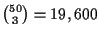. If there are k ranks larger than x, then the number of cards of rank larger than x is 4k which implies there are 50 - 4k cards of rank x or smaller. Thus, the number of flops not containing an overcard is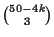. Dividing by 19,600 gives the probability of a flop with no overcards. Subtracting from 1 gives the probability of at least one overcard on the flop. For a pair of deuces there are zero flops not containing an overcard. For a pair of threes there are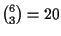flops not containing an overcard. For a pair of fours there are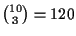flops without an overcard. For a pair of fives there are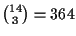flops without an overcard. For a pair of sixes there are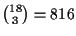flops without an overcard. For a pair of sevens there are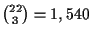flops without an overcard. For a pair of eights there areflops without an overcard. For a pair of nines there areflops without an overcard. For a pair of tens there are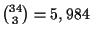flops without an overcard. For a pair of jacks there are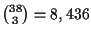flops without an overcard. For a pair of queens there areflops without an overcard. For a pair of kings there are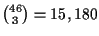flops without an overcard. For a pair of aces there are 19,600 flops without an overcard.

Subtracting each of the above values from 19,600 and dividing by 19,600 gives the probability of an overcard on the flop for a player holding a pocker pair of each rank. This is the probability listed in the column headed flop'' in Table 1 below.

We now repeat this for the board. There are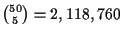possible boards for the player holding a pocket pair of rank x. The number of cards of rank x or smaller still is 50 - 4k using the same notation as above. So the number of boards not containing an overcard is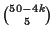. For a pair of deuces there are zero boards not containing an overcard. For a pair of threes there are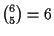boards not containing an overcard. For a pair of fours there are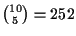boards without an overcard. For a pair of fives there are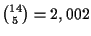boards without an overcard. For a pair of sixes there are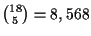boards without an overcard. For a pair of sevens there are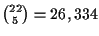boards without an overcard. For a pair of eights there are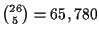boards without an overcard. For a pair of nines there are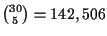boards without an overcard. For a pair of tens there are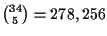boards without an overcard. For a pair of jacks there areboards without an overcard. For a pair of queens there areboards without an overcard. For a pair of kings there are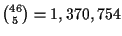boards without an overcard. For a pair of aces there are 2,118,760 boards without an overcard.

We subtract the preceding numbers from 2,118,760 and divide the result by 2,118,760 to get the probability of the board containing an overcard. These are the probabilities listed in the column headed board'' in Table 1.

 pair flop board 2,2 1.00 1.00 3,3 0.9990 0.999997 4,4 0.994 0.99988 5,5 0.981 0.9991 6,6 0.958 0.996 7,7 0.921 0.988 8,8 0.867 0.969 9,9 0.793 0.933 10,10 0.695 0.869 J,J 0.570 0.763 Q,Q 0.414 0.599 K,K 0.226 0.353 A,A 0.00 0.00

TABLE 1

The next topic we investigate is the probability a player holds a pocket pair of rank x and the flop contains either none, one, two or three cards of distinct larger rank, no pairs of larger rank and no more cards of rank x. We saw above that there are 19,600 flops in total. However, of these 19,600 flops, 48 contain a pair of rank x,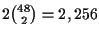contain one card of rank x, and the remaining 17,296 contain no card of rank x. We are interested in the probability of cards of bigger rank GIVEN that the player has not flopped any cards of rank x. Thus, we are working with the collection of 17,296 flops containing no cards of rank x.

When there are no pairs of bigger rank for a pair of deuces, then we must have three cards of distinct bigger rank on the flop. There are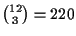choices for the ranks and four choices for each of the three ranks. This gives 14,080 flops. Dividing by 17,296 yields a probability of .814.

Now consider a pair of threes. The only way there can be no cards of bigger rank (remember we are assuming no threes have flopped) is if the flop consists of three deuces. Thus, there are four flops. If there is exactly one card of bigger rank, then there are 44 choices for the bigger card and the remaining two cards must be deuces. The latter may be chosen in six ways giving us 264 flops. If there are two cards of distinct bigger ranks, then there is one deuce, chosen in four ways, there are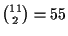choices for the big rank and four choices for each of the latter two ranks. This yields 3,520 flops. Finally, if there are three cards of bigger ranks and no pairs, there are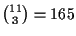choices for the ranks, and four choices for each card of a given rank. This yields 10,560 flops. Dividing the preceding numbers of flops by 17,296 gives the probabilities found in Table 2.

We move to pairs of fours. If the flop has no cards of larger rank, then there are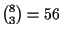flops because we are choosing the three cards from the eight deuces and treys. If there is one card of bigger rank, there are 40 choices for this card and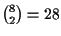choices for the two cards of ranks two and three. This produces 1,120 flops. For two cards of bigger ranks, there are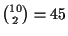choices for the two ranks, four choices for each card of a given rank, and eight choices for the single smaller rank card. The product gives 5,760 flops. Finally, for three bigger rank cards, there arechoices for the ranks and 64 choices for the three cards yielding 7,680 flops of this type. Dividing by 17,296 gives the entries of Table 2.

Consider pairs of fives. If the flop has no cards of larger rank, then we are choosing three cards from twelve giving us 220 flops. If there is one card of bigger rank, there are 36 choices for this card and 66 choices for the two smaller rank cards. The product is 2,376 which is the number of flops for one bigger rank card. For two cards of bigger ranks, there are 36 choices for the ranks, 16 choices for the two cards of these ranks, and 12 choices for the other card. Multiplying gives us 6,912 flops. For three cards of bigger ranks, there are 84 choices for the ranks and 64 choices for cards of those three ranks. We obtain 5,376 flops of this type. We get the corresponding probabilities in the usual way.

For a pair of sixes, there are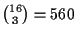flops with no cards of bigger rank. There are 32 choices for one card of bigger rank and 120 choices for two cards of smaller rank. Multiplying gives us 3,840 flops with precisely one card of bigger rank. There are 28 ways of choosing two larger ranks, 16 choices for two cards of those ranks, and 16 choices for one card of smaller rank. The number of flops is the product which is 7,168. Finally, there are 56 ways of choosing three larger ranks and 64 choices for cards of the chosen ranks. This gives us 3,584 flops with three cards of larger ranks with no pairs.

For a pair of sevens, there are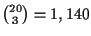flops with no cards of bigger rank. There are 28 choices for one card of bigger rank and 190 choices for two cards of smaller rank. Multiplying gives us 5,320 flops with precisely one card of bigger rank. There are 21 ways of choosing two larger ranks, 16 choices for two cards of those ranks, and 20 choices for one card of smaller rank. The number of flops is the product which is 6,720. Finally, there are 35 ways of choosing three larger ranks and 64 choices for cards of the chosen ranks. This gives us 2,240 flops with three cards of larger ranks with no pairs.

For a pair of eights, there are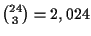flops with no cards of bigger rank. There are 24 choices for one card of bigger rank and 276 choices for two cards of smaller rank. Multiplying gives us 6,624 flops with precisely one card of bigger rank. There are 15 ways of choosing two larger ranks, 16 choices for two cards of those ranks, and 24 choices for one card of smaller rank. The number of flops is the product which is 5,760. Finally, there are 20 ways of choosing three larger ranks and 64 choices for cards of the chosen ranks. This gives us 1,280 flops with three cards of larger ranks with no pairs.

For a pair of nines, there are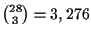flops with no cards of bigger rank. There are 20 choices for one card of bigger rank and 378 choices for two cards of smaller rank. Multiplying gives us 7,560 flops with precisely one card of bigger rank. There are 10 ways of choosing two larger ranks, 16 choices for two cards of those ranks, and 28 choices for one card of smaller rank. The number of flops is the product which is 4,480. Finally, there are 10 ways of choosing three larger ranks and 64 choices for cards of the chosen ranks. This gives us 640 flops with three cards of larger ranks with no pairs.

For a pair of tens, there are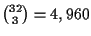flops with no cards of bigger rank. There are 16 choices for one card of bigger rank and 496 choices for two cards of smaller rank. Multiplying gives us 7,936 flops with precisely one card of bigger rank. There are six ways of choosing two larger ranks, 16 choices for two cards of those ranks, and 32 choices for one card of smaller rank. The number of flops is the product which is 3,072. Finally, there are 4 ways of choosing three larger ranks and 64 choices for cards of the chosen ranks. This gives us 256 flops with three cards of larger ranks with no pairs.

For a pair of jacks, there areflops with no cards of bigger rank. There are 12 choices for one card of bigger rank and 630 choices for two cards of smaller rank. Multiplying gives us 7,560 flops with precisely one card of bigger rank. There are three ways of choosing two larger ranks, 16 choices for two cards of those ranks, and 36 choices for one card of smaller rank. The number of flops is the product which is 1,728. Finally, there is one way of choosing three larger ranks and 64 choices for cards of the chosen ranks. This gives us 64 flops with three cards of larger ranks with no pairs.

For a pair of queens, there are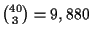flops with no cards of bigger rank (remember no queen either). There are eight choices for one card of larger rank and 780 choices for two cards of smaller ranks. This yields 6,240 flops of this type. There are 16 ways of choosing a king and an ace, and 40 choices for one card of smaller rank. Multiplying gives 640 flops with two unpaired cards of larger rank. It is not possible to have three cards of distinct larger ranks.

For a pair of kings, there areflops with no cards of bigger rank. There are four choices for an ace and 946 choices for two cards of smaller ranks. This gives 3,784 flops of this type. There cannot be two or three distinct larger ranks.

All 17,296 of the flops have no overcards for a pair of aces. We now are able to determine all entries of the next table. Recall that the probability under the column k larger'' is the probability of precisely k cards of larger rank, no two of which are paired, coming on the flop given that the player holding a pair of rank x does not flop any cards of rank x.

 pair 0 larger 1 larger 2 larger 3 larger 2,2 0.000 0.000 0.000 0.814 3,3 0.00023 0.015 0.204 0.611 4,4 0.0032 0.065 0.333 0.444 5,5 0.013 0.137 0.400 0.311 6,6 0.032 0.222 0.414 0.207 7,7 0.066 0.308 0.389 0.130 8,8 0.117 0.383 0.333 0.074 9,9 0.189 0.437 0.259 0.037 10,10 0.287 0.459 0.178 0.015 J,J 0.413 0.437 0.010 0.0037 Q,Q 0.571 0.361 0.037 0.000 K,K 0.766 0.219 0.000 0.000 A,A 1.00 0.000 0.000 0.000

TABLE 2

The last situation we consider in this file is when the player makes three-of-a-kind and a pair of bigger rank ends up on board as well. The player has a full house but the player's hand still is somewhat vulnerable. For this we consider both flops and boards.

Under the assumption the player has flopped three-of-a-kind, all we do is determine the probability the remaining two cards form a pair of bigger rank. When the pair is deuces and one deuce comes on the flop, there arechoices for the other two cards of the flop. Of these, 72 are a pair of bigger rank because there are 12 ranks and six pairs of each rank. This gives us a probability of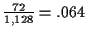that such an outcome occurs.

When the pair is threes and one three comes on the flop, there still are 1,128 choices for the other two cards, but now there are only 66 pairs of bigger rank. We get a probability of .059 for pocket threes.

For the remaining ranks for the pairs, the number 1,128 remains the same while the number of pairs decreases by six for each increase in rank of the pair. Finally, when we reach pocket aces, there are no pairs of bigger rank so we get a probability of 0. This information is included in the column headed by flop'' in Table 3.

We now want to do the same for boards. Again, we are assuming the player has one card on board of the rank of his pocket pair. The number of completions to form the board is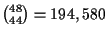in all cases. What we need to count is the number of ways of choosing four cards from the 48 so that the board ends up with either a single pair whose rank is bigger than the player's pocket pair, or the board ends up with two pair at least one of which has rank bigger than the player's pocket pair.

We start with pocket deuces. There arechoices of three ranks, three choices for the rank of the pair, six choices for the pair, and four choices for each of the cards of the other two ranks. The product is 63,360 and this is the number of completions to a board with a single pair of bigger rank. The number of completions with two pair is 66 for the ranks of the pairs multiplied by six for each pair giving 2,376 choices of four cards. This gives us 65,736 choices of four cards and dividing by 194,580 yields a probability of .338 in this case.

For pocket threes in the case of a single pair, there are 11 choices for the rank of the pair, six choices for the pair,choices for the other two ranks, and four choices for each of the cards. This product is 58,080. When there are two pair on board, one of them must have rank bigger than three so we need count only all the choices of four cards giving two pair. This is the same as the preceding case, that is, 2,376. The sum is 60,456 and dividing by 194,580 gives a probability of .311 for pocket threes.

For pocket fours in the case of a single pair, there are 10 choices for the rank of the pair, six choices for the pair,choices for the other two ranks, and four choices for each of the cards. This product is 52,800. As before, there are 2,376 choices of four cards resulting in two pair on board. The only case not qualifying is if the two pair are deuces and treys which can happen in 36 ways. Thus, 2,340 of the two pair have at least one pair with rank bigger than four. The sum is 55,140 and dividing by 194,580 gives a probability of .283 for pocket fours.

For pocket fives in the case of a single pair, there are nine choices for the rank of the pair, six choices for the pair,choices for the other two ranks, and four choices for each of the cards. This product is 47,520. As before, there are 2,376 choices of four cards resulting in two pair on board. The cases not qualifying are for two pair with both ranks smaller than five. There are three combinations of smaller ranks with 36 choices for each combination. Removing 108 from 2,376 leaves 2,268 two pairs having at least one pair with rank bigger than five. The sum is 49,788 and dividing by 194,580 gives a probability of .256 for pocket fives.

For pocket sixes in the case of a single pair, there are eight choices for the rank of the pair, six choices for the pair,choices for the other two ranks, and four choices for each of the cards. This product is 42,240. As before, there are 2,376 choices of four cards resulting in two pair on board. The cases not qualifying are for two pair with both ranks smaller than six. There are six combinations of smaller ranks with 36 choices for each combination. Removing 216 from 2,376 leaves 2,160 two pairs having at least one pair with rank bigger than six. The sum is 44,400 and dividing by 194,580 gives a probability of .228 for pocket sixes.

For pocket sevens in the case of a single pair, there are seven choices for the rank of the pair, six choices for the pair,choices for the other two ranks, and four choices for each of the cards. This product is 36,960. As before, there are 2,376 choices of four cards resulting in two pair on board. The cases not qualifying are for two pair with both ranks smaller than seven. There are ten combinations of smaller ranks with 36 choices for each combination. Removing 360 from 2,376 leaves 2,016 two pairs having at least one pair with rank bigger than seven. The sum is 38,976 and dividing by 194,580 gives a probability of .200 for pocket sevens.

For pocket eights in the case of a single pair, there are six choices for the rank of the pair, six choices for the pair,choices for the other two ranks, and four choices for each of the cards. This product is 31,680. As before, there are 2,376 choices of four cards resulting in two pair on board. The cases not qualifying are for two pair with both ranks smaller than eight. There are 15 combinations of smaller ranks with 36 choices for each combination. Removing 540 from 2,376 leaves 1,836 two pairs having at least one pair with rank bigger than eight. The sum is 33,536 and dividing by 194,580 gives a probability of .172 for pocket eights.

For pocket nines in the case of a single pair, there are five choices for the rank of the pair, six choices for the pair,choices for the other two ranks, and four choices for each of the cards. This product is 26,400. As before, there are 2,376 choices of four cards resulting in two pair on board. The cases not qualifying are for two pair with both ranks smaller than nine. There are 21 combinations of smaller ranks with 36 choices for each combination. Removing 756 from 2,376 leaves 1,620 two pairs having at least one pair with rank bigger than nine. The sum is 28,020 and dividing by 194,580 gives a probability of .144 for pocket nines.

For pocket tens in the case of a single pair, there are four choices for the rank of the pair, six choices for the pair,choices for the other two ranks, and four choices for each of the cards. This product is 21,120. As before, there are 2,376 choices of four cards resulting in two pair on board. The cases not qualifying are for two pair with both ranks smaller than ten. There are 28 combinations of smaller ranks with 36 choices for each combination. Removing 1,008 from 2,376 leaves 1,368 two pairs having at least one pair with rank bigger than ten. The sum is 22,488 and dividing by 194,580 gives a probability of .144 for pocket tens.

For pocket jacks in the case of a single pair, there are three choices for the rank of the pair, six choices for the pair,choices for the other two ranks, and four choices for each of the cards. This product is 15,840. As before, there are 2,376 choices of four cards resulting in two pair on board. The cases not qualifying are for two pair with both ranks smaller than jack. There are 36 combinations of smaller ranks with 36 choices for each combination. Removing 1,296 from 2,376 leaves 1,080 two pairs having at least one pair with rank bigger than jack. The sum is 16,920 and dividing by 194,580 gives a probability of .087 for pocket jacks.

For pocket queens in the case of a single pair, there are two choices for the rank of the pair, six choices for the pair,choices for the other two ranks, and four choices for each of the cards. This product is 10,560. As before, there are 2,376 choices of four cards resulting in two pair on board. The cases not qualifying are for two pair with both ranks smaller than queen. There are 45 combinations of smaller ranks with 36 choices for each combination. Removing 1,620 from 2,376 leaves 756 two pairs having at least one pair with rank bigger than queen. The sum is 11,316 and dividing by 194,580 gives a probability of .058 for pocket queens.

For pocket kings in the case of a single pair, there is one choice for the rank of the pair, six choices for the pair,choices for the other two ranks, and four choices for each of the cards. This product is 5,280. As before, there are 2,376 choices of four cards resulting in two pair on board. The cases not qualifying are for two pair with both ranks smaller than king. There are 55 combinations of smaller ranks with 36 choices for each combination. Removing 1,980 from 2,376 leaves 396 two pairs having at least one pair with rank bigger than king. The sum is 5,676 and dividing by 194,580 gives a probability of .029 for pocket kings.

For pocket aces there are no pairs of bigger rank. We now have the numbers for Table 3 below. We reiterate what information the table contains. Given that a player is holding a pair of rank x in his hand, the column headed flop'' gives the probability a pair of rank bigger than x comes on the flop given that one card of rank x is in the flop. The column headed board'' gives the probability there is at least one pair of rank bigger than xon board given that one card of rank x is on board but neither trips nor quads are on board.

 pair flop board 2,2 0.064 0.338 3,3 0.059 0.311 4,4 0.053 0.283 5,5 0.048 0.256 6,6 0.043 0.228 7,7 0.037 0.200 8,8 0.032 0.172 9,9 0.027 0.144 10,10 0.021 0.116 J,J 0.016 0.087 Q,Q 0.011 0.058 K,K 0.005 0.029 A,A 0.000 0.000

TABLE 3

Home | Publications | Preprints | MITACS | Poker Digest | Poker Computations | Feedback
website by the Centre for Systems Science
last updated 12 January 2000